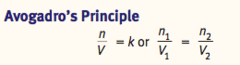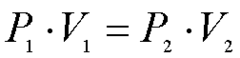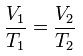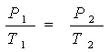# Physical Chemistry Chapter 8 (Gas)Patricia Harrah
question

*8.1 Gas Phase*

question

General characteristics of gases

Atoms move rapidly and are far apart. Only weak intermolecular forces exist between gas particles – therefore they will fill any volume. They are easily compressible.
question

Define by what variables?

Pressure, volume, temperature, moles
question

Pressure units

1 atm = 760 Torr = 760 mmHg = 101.325 kPa
question

Medical devices that measure blood pressure, and how do they work?

Sphygomomanometers => sphygo (pulse) – manometer (measure pressure) The cuff creates a force that is OPPOSED by the person’s systolic and diastolic arterial blood pressure.
question

How does a simple barometer work?

Atmospheric pressure creates downward force on pool of mercury. Mercury in column exerts opposing force based on its density. Weight of mercury creates vacuum in top of tube. When external air exerts higher force than weight of mercury in column, column rises. Reading in mmHg is directly proportional to atmospheric pressure being applied.
question

Standard temperature and pressure (gases)

1 atm and 0 Celsius (273K) THIS IS DIFFERENT THAN STANDARD STATE (25 degrees, 1 atm, 1M)
question

*8.2 Ideal Gases*

question

Ideal gas

Hypothetical gas with molecules that have no intermolecular forces and occupy no volume.
question

Real gases

Deviate from ideal behavior at high pressure and low temperatures. But many compressed gases act roughly ideal.
question

Ideal gas law

PV = nRT R = 8.21 x 10^2 (may be given in other [email protected]#)
question

Density

mass per volume.
question

Rewrite the ideal gas law for the density of gas

PV = nRT where n = m/M (mass over molar mass) PV = (m/M)RT m/V = PM/RT
question

Mole of ideal gas at STP =

22.4 L
question

Combined law

Used to relate conditions at STP to conditions of actually temperature and pressure. ASSUMES that number of moles stays constant, therefore you can set two PV/T terms equal to each other.
question

Rearrangements of combined law to find volume and density under nonstandard conditions

Rearrange to get V2. V2 = V1[P1/P2][T2/T1] then do rho = m/V2
question

Experimentally find molar mass

Measure pressure and temp. of a gas in a bulb of a given volume (we get P, T, V). Remove gas from bulb, and determine mass of sample (mass of bulb with sample minus mass of empty bulb). can get density, and then multiply density by 22.4 L/mol
question

Do the question on page 256 it’s messed up

questionAll gases at constant temperature and pressure occupy volumes that are directly proportional to the moles of gas present. One mole of any gas will occupy 22.4L at STP
question

Boyle’s LawFor a given gaseous sample held at constant temperature, volume of a gas is inversely proportional to its pressure. PV = k or P1V1 = P2V2
question

Charles’s LawAt constant pressure, volume of a gas is proportional to its absolute temperature in kelvins V/T = k or V1/T1 = V2/T2
question

Gay-Lussac’s LawRelates pressure to temperature.
question

How to do all these questions

Just use the combined gas law and cancel out the terms that are constant. Combined gas law cmobines Boyle’s law, Charle’s law and Gay-Lussac’s law. Just need to remember Avogardo’s.
question

Partial pressure

Pressure exerted by each individual gas. If two or more gases don’t chemically interact, each gas in the vessel behaves as if it were the only gas in the container -> exerts the same pressure it would if it were on its own.
question

Dalton’s law of partial pressures

Total pressure of gaseous mixture equal to sum of partial pressures.
question

Partial pressure related to its mole fraction

X = moles of one gas species/total moles of gas Find the fraction of total moles the moles of gas of interest represents, then multiply that fraction by the total pressure to get the partial pressure
question

Henry’s law

Based on observation that at various applied pressure, [gas] in a liquid increased or decreased. Vapor pressure from evaporated molecules above the surface of a liquid forces some of the gas back into the liquid phase – equilibrium reached between evaporation and condensation. [A] = kh x Pa [A]1/P1 = [A]2/P2 = kh where kh is Henry’s constant, Pa is partial pressure A. Value of kh depends on gas. This means that solubility (concentration) and pressure are directly related
question

Application of Henry’s law

For the lungs, if atmospheric pressure changes then the partial pressure of oxygen in the atmosphere changes and the amount of the gas exchanged is altered. e.g. If partial pressure is elevated, the amount of that gas dissolved in the blood is also elevated.
question

*8.3 Kinetic Molecular Theory*

question

Assumptions of Kinetic Molecular Theory

1. Gases made up of particles with negligible volumes 2. Gas atoms/molecules exhibit no intermolecular interactions 3. Gas particles in continuous random motion, and undergoing collisions. 4. Collisions between two any gas particles or between particles and container are ELASTIC (conservation of momentum and KE) 5. Average KE of gas particles proportional to absolute temperature of gas in kelvin, and is equal for all gases at a given temperature.
question

Average KE of gas particle equation

KE = 1/2 mv^2 = 3/2KbT where Kb is the Boltzmann constant. Speed of gas related to absolute temperature. *This only gves the average molecular speed* i.e. the higher the temperature, the faster the particles move
question

Root-mean-square speed

Average kinetic energy per particle –> calculate speed urms = aqrt(3RT/M) i.e. the larger the molecules, the slower they move
question

Maxwell-Boltzmann distribution curve

Shows distribution of gas praticle speeds at given temperature. As temp increases, curve flattens are shifts to the right.
question

Graham’s Law

Heavier gases diffuse more slowly than lighter ones because of their differing average speeds. Because all gas particles have SAME AVERAGE KE AT THE SAME TEMP, then it must be true that particles with greater mass travel more slowly on average (ke = 1/2 m*v^2)
question

Effusion

Flow of gas particles under pressure from one compartment to another through a small opening. For two gases at the same temperature, rates of effusion are proportional to the average speeds. Therefore heavier gases will effuse at a decreased rate.
question

*8.4 Real Gases*

question

Real gases characteristics

Particles occupy non-negligible volumes and interact with each other in measurable waves. However, real gases act like ideal gases when compressed (high pressure) or at low temperature.
question

Real gases deviations due to pressure

As pressure of gas increases, particles are pushed closer together. Intermolecular attraction forces become more and more significant, and gas condenses to liquid. At moderately high pressure, volume is less than would be predicted by PV = nRT because of intermolecular attraction. At extremely high pressures, size of particles becomes relatively larger than the distance between particles, causing gas to take up a larger volume than would be predicted by PV = nRT (which would predict that gas could be compressed to take up 0 volume)
question

Real gases deviations due to temperature